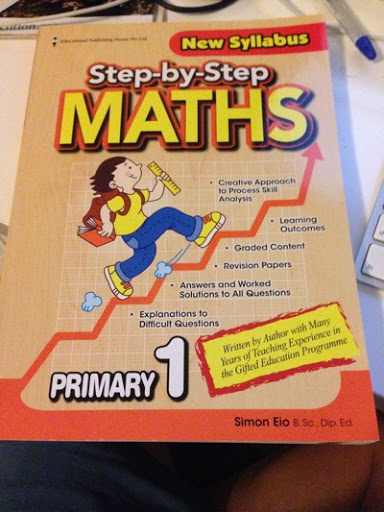# The Singapore Model Method for Learning Mathematics

Date:  Mar 4, 2013

Call me a Kia-su Parent, I don’t care!  But what I care is, when your kid bring a simple math question (or is it an English question about math) to you and you are not able to solve it using the correct Singapore way.  What will you do?  Will it be too late to learn the correct math?

Welcome to the world of “Model Method” for learning Mathematics.  🙂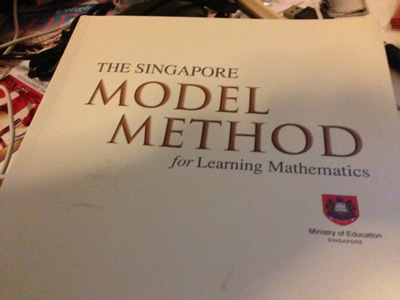From time to time, my ex-colleagues will pass me some math questions for their primary kids and hope that I, “the Engineer” can solve it.  Well, the truth is, when I see the question, it is very simple question.  But when you try to solve it, by nature, from what we learned 30 years ago… we used Algebra to solve it. In fact, most of the questions took me more than 10 minutes to solve.

Wait now, we are talking about primary school children, so, how on earth that they know about Algebra in Primary school?

Well, Singapore’s answer is the “Model Method”.  A very powerful visual method to help solve complicated math question for Primary School students.

I know Jay Jay is only Primary 1 this year.  And they only learn about Model method in P3 onwards.  But you know me, I have strong interest to learn and explore “Model Method” and there I go…

To sign on a one day short seminar about Model Method.  From http://OnSponge.com

(Note:  You don’t really have to pay money to go for this seminar, some school do provide this free or as low as \$2 with free food.)The reason I went is because one of my friend went there, and she think it is good.

Also, these are the people who write math books for schools here in Singapore.  Only sell it in school’s book store I heard.  So, can’t be wrong right?

I bought this book after the lesson so that I can practise 3 questions a week.  So, by the time Jay Jay reached P3, his daddy will be or should be able to solve any kind of math questions using the Model method. kekekeOK.  Let’s do one simple question so that we can have a quick peep on what is Model Method.

Question:  There were 320 more red marbles than blue marbles in a bag.  If 30 red marbles were removed and 80 blue marbles were added, there were thrice as many red marbles as blue marbles in the bag.  How many marbles were there in the bag???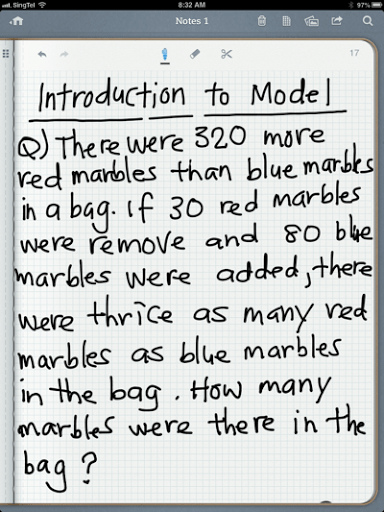To solve this question, one has to be GOOD in ENGLISH.

Also, one has to be able to brackets or highlights the different condition.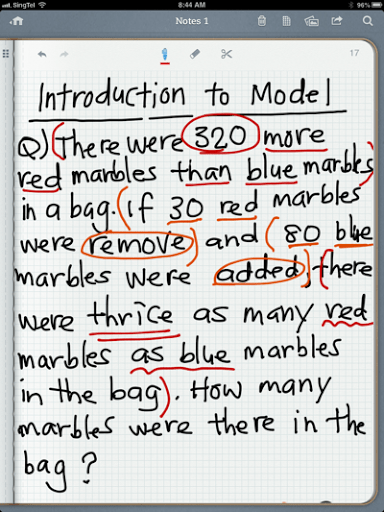Once you have understand the question.  It is time to draw the model.

So, there are 320 more red marbles than the blue one.  So, you draw two blocks, one for red and the other for blue.

Stacks both blocks together.  And then, the red one should have 320 more marbles.  So, you indicate “320” in the additional block for red.  At this stage, you don’t know how many blue marbles, or whether it is more than 320 or lesser.  So the SIZE of block is not important.  So, don’t worry about the proportion of the blocks.Now, some thing has happened.  So, you drew the same model diagram below and call it “IF (this happen)”.

So, what has happened?  IF 30 red one were removed…. So, you SHADE the small block and label it 30.  Whenever you SHADE the block, that means, you have remove that number of block from the red one.  In our example, 30 red one is removed.

Very important tip is to use pencil to draw the model.  Because from time to time, you will need to erase the block and redraw so that it is nicer and easy to understand.Next, 80 blue marbles are added.  So, you draw a small incremental block beside the blue block.  In our case, label “80” on it.  This is to indicate that you added 80 blue marbles.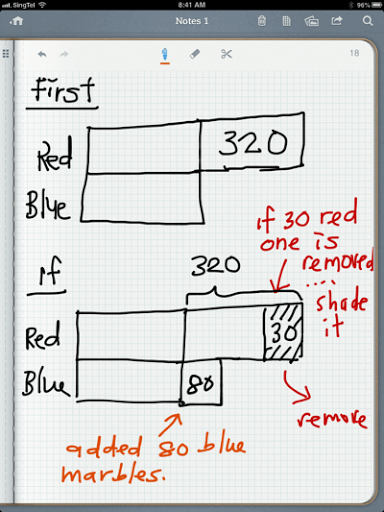Next step.  “EQUAL PARTS”.  So, if you add 80 down at the blue block.  You EXTEND a line in the red block.  Then, fill in “80”.  This is call to EQUAL the PARTS.

Now, you now that the additional block beside the red original unlabelled block has a TOTAL of 320.  So, that makes the remaining parts to be 210.  i.e. 320 – 30 – 80 = 210.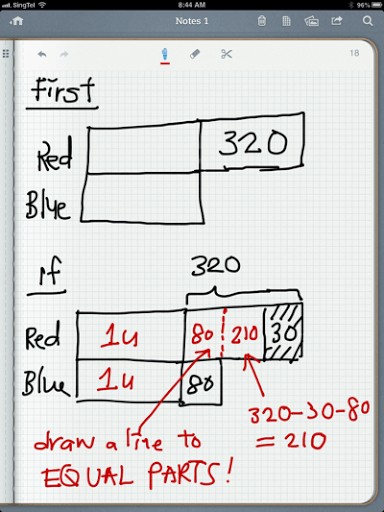Lastly, “There are thrice as many red marbles as blue marbles at the end.  So, the total red block represents 3 UNITS compare to 1 UNIT of blue block.

So, the remaining 2 units is actually 210.  Can you see it?  The original 1u blue + 80 = 1 unit of blue.  So, the total red now becomes three times of this 1 unit of blue.  So, that makes 210 equal to 2 unit of blue.  So, solving the 2 unit of blue will tell you what is a single unit.

As you can see … the confusing part is the size of the block of 210 is almost equal to size of 80.  And the 1u size is greater than these 80 and 210 blocks.  So, that is why proportions are not important.  The key important concept is that you know how to visualise the model diagram and solve the puzzle.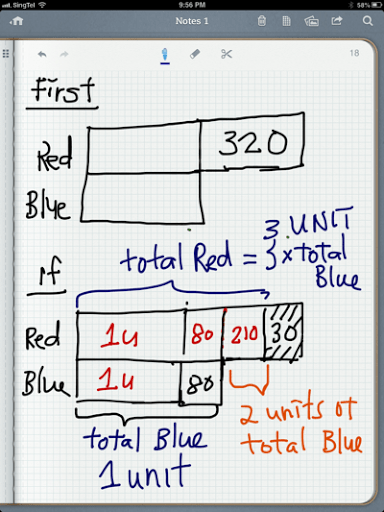Once you can see 2 units of blue equal 210.  You can easily solve the math question.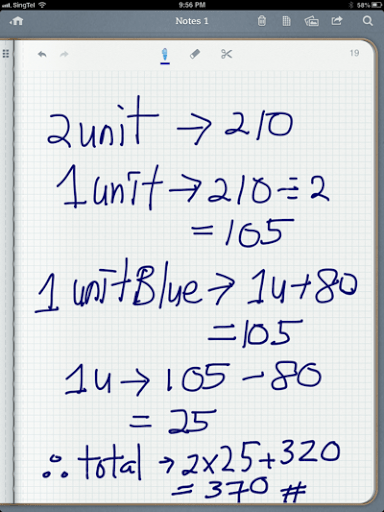The truth is, when I first read the questions, I got stuck there for almost 2-3 minutes with my mind blank.  But after the trainer explain how you solve it, I am like understand a bit here and there.

Then, when I continue the next 15 questions in different categories, again, each question is like a new question to me.  So, it is not easy.  And I am glad that I went to course to learn this.

At least, it gives me an idea what I am facing in next two years.  And it also gives me the preparation I need to teach my Jay Jay the correct math.  So, it is important to teach them to think how to solve it using model diagram.  No longer you need to memorise formulas or solve complicated algebra.  By using some block diagrams, you are able to solve these math questions.It is a big WOW for me, but I enjoyed every single questions and every single challenge.

This is another good book where the trainer heard it is good.  This is for P1.